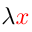Higher-Order Logic Explorer < Previous   Next > Nearby theorems Mirrors  >  Home  >  HOLE Home  >  Th. List  >  ded GIF version

Theorem ded 74
 Description: Deduction theorem for equality.
Hypotheses
Ref Expression
ded.1 (R, S)⊧T
ded.2 (R, T)⊧S
Assertion
Ref Expression
ded R⊧[S = T]

Proof of Theorem ded
StepHypRef Expression
1 weq 38 . 2 = :(∗ → (∗ → ∗))
2 ded.2 . . 3 (R, T)⊧S
32ax-cb2 30 . 2 S:∗
4 ded.1 . . 3 (R, S)⊧T
54ax-cb2 30 . 2 T:∗
64, 2ax-ded 43 . 2 R⊧(( = S)T)
71, 3, 5, 6dfov2 67 1 R⊧[S = T]
 Colors of variables: type var term Syntax hints:  ∗hb 3   = ke 7  [kbr 9  kct 10  ⊧wffMMJ2 11 This theorem was proved from axioms:  ax-syl 15  ax-jca 17  ax-trud 26  ax-cb1 29  ax-cb2 30  ax-refl 39  ax-eqmp 42  ax-ded 43  ax-ceq 46 This theorem depends on definitions:  df-ov 65 This theorem is referenced by:  dedi  75  eqtru  76  ex  148  notval2  149  dfex2  185
 Copyright terms: Public domain W3C validator